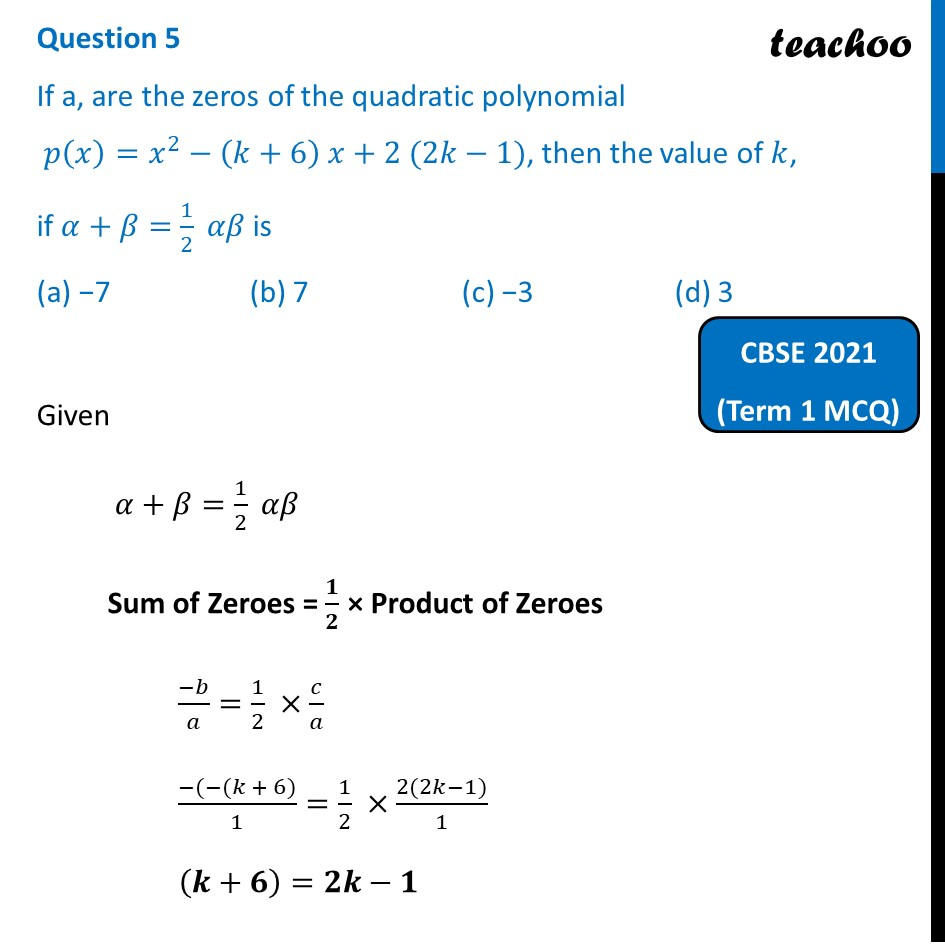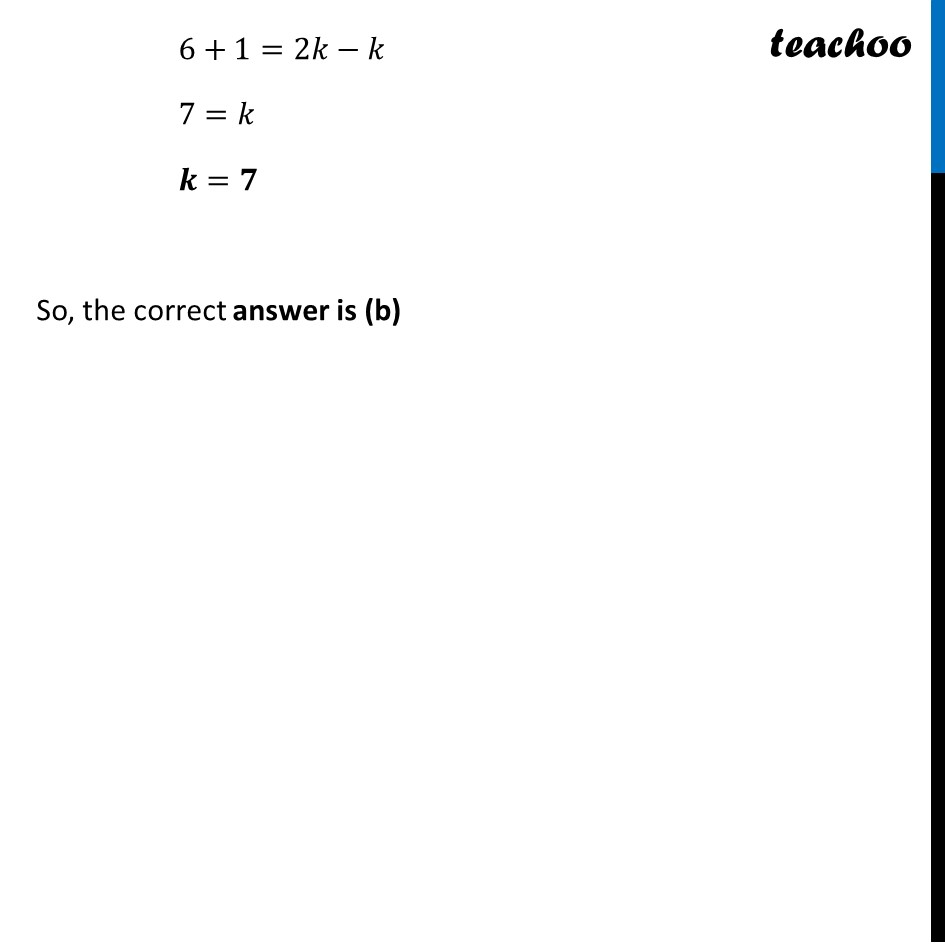Past Year MCQ (Maths Standard)

Chapter 2 Class 10 Polynomials
Serial order wise

## (d) 3Learn in your speed, with individual attention - Teachoo Maths 1-on-1 Class

### Transcript

Question 5 If a, are the zeros of the quadratic polynomial 𝑝(𝑥)=𝑥^2−(𝑘+6) 𝑥+2 (2𝑘−1), then the value of 𝑘, if 𝛼+𝛽=1/2 𝛼𝛽 is (a) −7 (b) 7 (c) −3 (d) 3 Given 𝛼+𝛽=1/2 𝛼𝛽 Sum of Zeroes = 𝟏/𝟐 × Product of Zeroes (−𝑏)/𝑎=1/2 ×𝑐/𝑎 (−(−(𝑘 + 6))/1=1/2 ×(2(2𝑘−1))/1 (𝒌+𝟔)=𝟐𝒌−𝟏 6+1=2𝑘−𝑘 7=𝑘 𝒌=𝟕 So, the correct answer is (b)